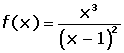Learn from home

The teachersChapters

At an inflection point, the function is not concave or convex but is changing from concavity to convexity or vice versa.

If f and f' are differentiable at a.### Calculation of the Points of Inflection

Calculate the inflection points of:

f(x) = x³ − 3x + 2

To find the inflection points, follow these steps:

1. Find the second derivative and calculate its roots.

f''(x) = 6x 6x = 0 x = 0.

2. Determine the 3rd derivative and calculate the sign that the zeros take from the second derivative and if:

f'''(x) ≠ 0 There is an inflection point.

f'''(x) = 6 It is an inflection point.

3. Calculate the image (in the function) of the point of inflection.

f(0) = (0)³ − 3(0) + 2 = 2

Inflection point: (0, 2)

## Example

Calculate the points of inflection of the function:Domain:There is an inflection point at x = 0, since the function changes from concave to convex.Inflection point (0, 0)

Did you like the article?(1 votes, average: 5.00 out of 5)Loading...

Emma

I am passionate about travelling and currently live and work in Paris. I like to spend my time reading, gardening, running, learning languages and exploring new places.

Did you like
this resource?

Bravo!